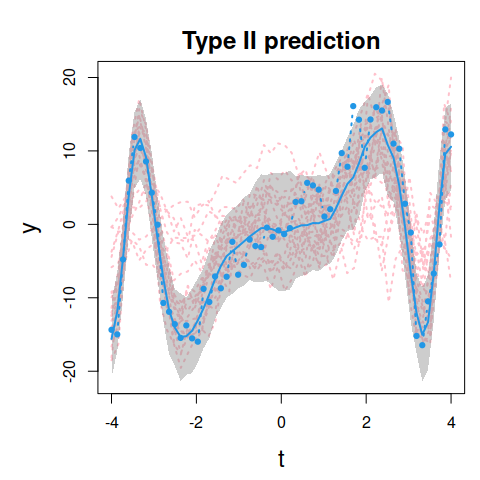# GPFR example

Suppose we have a functional response variable $$y_m(t), \ m=1,\dots,M$$, a functional covariate $$x_m(t)$$ and also a set of $$p=2$$ scalar covariates $$\textbf{u}_m = (u_{m0},u_{m1})^\top$$.

A Gaussian process functional regression (GPFR) model used in this example is defined by

$$y_m(t) = \mu_m(t) + \tau_m(x_m(t)) + \varepsilon_m(t)$$,

where $$\mu_m(t) = \textbf{u}_m^\top \boldsymbol{\beta}(t)$$ is the mean function model across different curves and $$\tau_m(x_m(t))$$ is a Gaussian process with zero mean and covariance function $$k_m(\boldsymbol{\theta}|x_m(t))$$. That is, $$\tau_m(x_m(t))$$ defines the covariance structure of $$y_m(t)$$ for the different data points within the same curve.

The error term can be assumed to be $$\varepsilon_m(t) \sim N(0, \sigma_\varepsilon^2)$$, where the noise variance $$\sigma_\varepsilon^2$$ can be estimated as a hyperparameter of the Gaussian process.

In the example below, the training data consist of $$M=20$$ realisations on $$[-4,4]$$ with $$n=50$$ points for each curve. We assume regression coefficient functions $$\beta_0(t)=1$$, $$\beta_1(t)=\sin((0.5 t)^3)$$, scalar covariates $$u_{m0} \sim N(0,1)$$ and $$u_{m1} \sim N(10,5^2)$$ and a functional covariate $$x_m(t) = \exp(t) + v$$, where $$v \sim N(0, 0.1^2)$$. The term $$\tau_m(x_m(t))$$ is a zero mean Gaussian process with exponential covariance kernel and $$\sigma_\varepsilon^2 = 1$$.

We also simulate an $$(M+1)$$th realisation which is used to assess predictions obtained by the model estimated by using the training data of size $$M$$. The $$y_{M+1}(t)$$ and $$x_{M+1}(t)$$ curves are observed on equally spaced $$60$$ time points on $$[-4,4]$$.

library(GPFDA)
require(MASS)
set.seed(100)

M <- 20
n <- 50
p <- 2  # number of scalar covariates

hp <- list('pow.ex.v'=log(10), 'pow.ex.w'=log(1),'vv'=log(1))

## Training data: M realisations -----------------

tt <- seq(-4,4,len=n)
b <- sin((0.5*tt)^3)

scalar_train <- matrix(NA, M, p)
t_train <- matrix(NA, M, n)
x_train <- matrix(NA, M, n)
response_train <- matrix(NA, M, n)
for(i in 1:M){
u0 <- rnorm(1)
u1 <- rnorm(1,10,5)
x <- exp(tt) + rnorm(n, 0, 0.1)
Sigma <- cov.pow.ex(hyper = hp, input = x, gamma = 1)
diag(Sigma) <- diag(Sigma) + exp(hp$vv) y <- u0+u1*b + mvrnorm(n=1, mu=rep(0,n), Sigma=Sigma) scalar_train[i,] <- c(u0,u1) t_train[i,] <- tt x_train[i,] <- x response_train[i,] <- y } ## Test data (M+1)-th realisation ------------------ n_new <- 60 t_new <- seq(-4,4,len=n_new) b_new <- sin((0.5*t_new)^3) u0_new <- rnorm(1) u1_new <- rnorm(1,10,5) scalar_new <- cbind(u0_new, u1_new) x_new <- exp(t_new) + rnorm(n_new, 0, 0.1) Sigma_new <- cov.pow.ex(hyper = hp, input = x_new, gamma = 1) diag(Sigma_new) <- diag(Sigma_new) + exp(hp$vv)
response_new <- u0_new + u1_new*b_new + mvrnorm(n=1, mu=rep(0,n_new),
Sigma=Sigma_new)

The estimation of mean and covariance functions in the GPFR model is done using the gpfr function:

a1 <- gpfr(response = response_train, time = tt, uReg = scalar_train,
fxReg = NULL, gpReg = x_train,
fyList = list(nbasis = 23, lambda = 0.0001),
uCoefList = list(list(lambda = 0.0001, nbasi = 23)),
Cov = 'pow.ex', gamma = 1, fitting = T)

Note that the estimated covariance function hyperparameters are similar to the true values:

unlist(lapply(a1\$hyper,exp))
#> pow.ex.v pow.ex.w       vv
#>  10.8033   0.8482   1.2198

### Plot of raw data

To visualise all the realisations of the training data:

plot(a1, type='raw')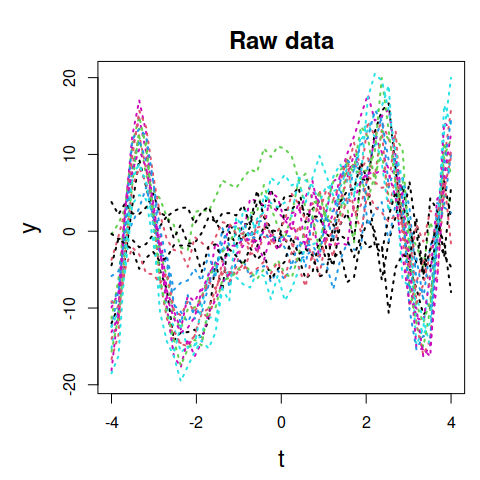To visualise three realisations of the training data:

plot(a1, type='raw', realisations = 1:3)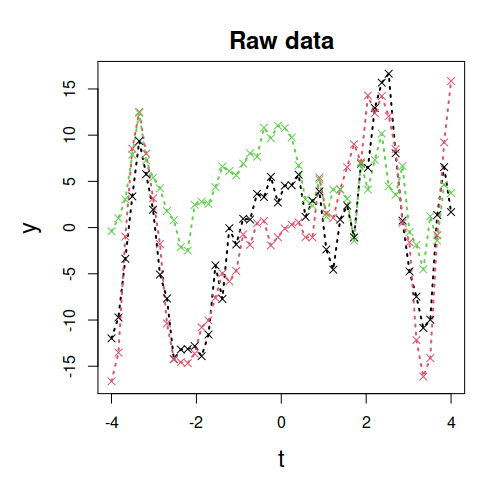### FR fit for training data

The in-sample fit using mean function from FR model only can be seen:

plot(a1, type = 'meanFunction', realisations = 1:3)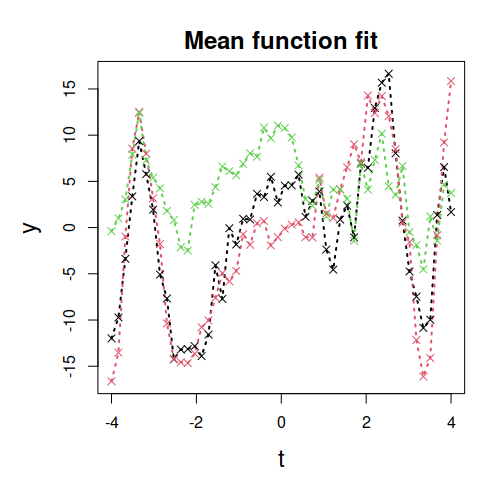### GPFR fit for training data

The GPFR model fit to the training data is visualised by using:

plot(a1, type = 'fitted', realisations = 1:3)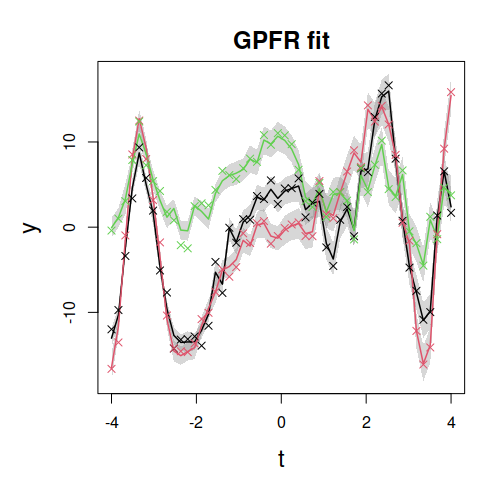### Type I prediction: $$y_{M+1}$$ observed

If $$y_{M+1}(t)$$ is observed over all the domain of $$t$$, the Type I prediction can be seen:

b1 <- gpfrPredict(a1, testInputGP = x_new, testTime = t_new,
uReg = scalar_new, fxReg = NULL,
gpReg = list('response' = response_new,
'input' = x_new,
'time' = t_new))

plot(b1, type = 'prediction', colourTrain = 'pink')
lines(t_new, response_new, type = 'b', col = 4, pch = 19, cex = 0.6, lty = 3, lwd = 2)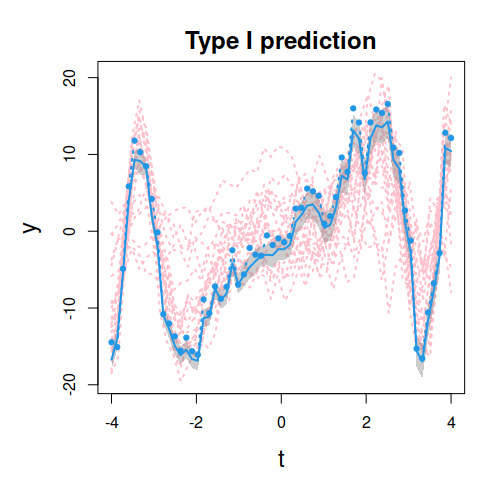### Type I prediction: $$y_{M+1}$$ partially observed

If we assume that $$y_{M+1}(t)$$ is only partially observed, we can obtain Type I predictions via:

b2 <- gpfrPredict(a1, testInputGP = x_new, testTime = t_new,
uReg = scalar_new, fxReg = NULL,
gpReg = list('response' = response_new[1:20],
'input' = x_new[1:20],
'time' = t_new[1:20]))

plot(b2, type = 'prediction', colourTrain = 'pink')
lines(t_new, response_new, type = 'b', col = 4, pch = 19, cex = 0.6, lty = 3, lwd = 2)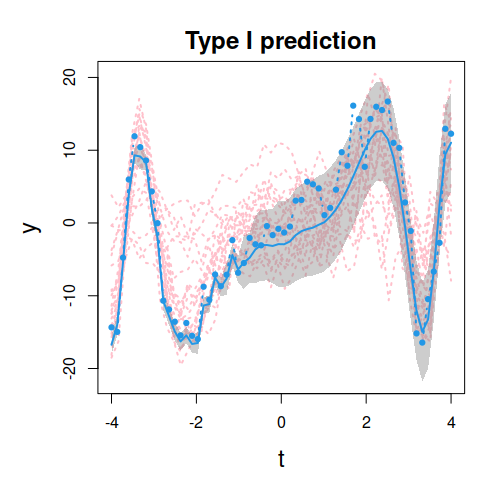### Type II prediction: $$y_{M+1}$$ not observed

Type II prediction, which is made by not including any information about $$y_{M+1}(t)$$, is visualised below.

b3 <- gpfrPredict(a1, testInputGP = x_new, testTime = t_new,
uReg = scalar_new, fxReg = NULL, gpReg = NULL)

plot(b3, type = 'prediction', colourTrain = 'pink')
lines(t_new, response_new, type='b', col = 4, pch = 19, cex = 0.6, lty = 3, lwd = 2)